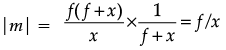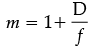NEET  >  Assertion & Reason Test: Ray Optics & Optical Instruments - 1

# Assertion & Reason Test: Ray Optics & Optical Instruments - 1

Test Description

## 10 Questions MCQ Test Physics Class 12 | Assertion & Reason Test: Ray Optics & Optical Instruments - 1

Assertion & Reason Test: Ray Optics & Optical Instruments - 1 for NEET 2022 is part of Physics Class 12 preparation. The Assertion & Reason Test: Ray Optics & Optical Instruments - 1 questions and answers have been prepared according to the NEET exam syllabus.The Assertion & Reason Test: Ray Optics & Optical Instruments - 1 MCQs are made for NEET 2022 Exam. Find important definitions, questions, notes, meanings, examples, exercises, MCQs and online tests for Assertion & Reason Test: Ray Optics & Optical Instruments - 1 below.
Solutions of Assertion & Reason Test: Ray Optics & Optical Instruments - 1 questions in English are available as part of our Physics Class 12 for NEET & Assertion & Reason Test: Ray Optics & Optical Instruments - 1 solutions in Hindi for Physics Class 12 course. Download more important topics, notes, lectures and mock test series for NEET Exam by signing up for free. Attempt Assertion & Reason Test: Ray Optics & Optical Instruments - 1 | 10 questions in 20 minutes | Mock test for NEET preparation | Free important questions MCQ to study Physics Class 12 for NEET Exam | Download free PDF with solutions
 1 Crore+ students have signed up on EduRev. Have you?
Assertion & Reason Test: Ray Optics & Optical Instruments - 1 - Question 1

### Directions : In the following questions, A statement of Assertion (A) is followed by a statement of Reason (R). Mark the correct choice as.Assertion (A): The focal length of a concave mirror is f and an object is placed at a distance x from the focus. The magnification produced by the mirror is f/x.Reason (R): magnification = size of image / size of object

Detailed Solution for Assertion & Reason Test: Ray Optics & Optical Instruments - 1 - Question 1 u = f+x

Using mirror formula,

1/v+1/u = 1/f

Or, 1/v – 1/(f+x) = – 1/f

∴ v = – f(f+x)/x

Magnification (m) = size of image / size of object = – v/uSo, the assertion and reason both are true and the reason properly explains the assertion.

Assertion & Reason Test: Ray Optics & Optical Instruments - 1 - Question 2

### Directions : In the following questions, A statement of Assertion (A) is followed by a statement of Reason (R). Mark the correct choice as.Assertion (A): Air bubbles shine in water.Reason (R): Air bubbles shine in water due to refraction of light.

Detailed Solution for Assertion & Reason Test: Ray Optics & Optical Instruments - 1 - Question 2 Air bubbles shine in water due to total internal reflection. Total internal reflection is a special case of refraction when light passes from denser medium (water) to rarer medium (air inside the bubble) and angle of incidence is more than the critical angle. So, the reason is also true. The reason also explains the assertion properly.
Assertion & Reason Test: Ray Optics & Optical Instruments - 1 - Question 3

### Directions : In the following questions, A statement of Assertion (A) is followed by a statement of Reason (R). Mark the correct choice as.Assertion (A): A double convex air bubble is formed within a glass slab. The air bubble behaves like a converging lens.Reason (R): Refractive index of glass is more than the refractive index of air.

Detailed Solution for Assertion & Reason Test: Ray Optics & Optical Instruments - 1 - Question 3 Speed of light is slower in glass compared to that in air. Hence the refractive index of glass is more than that of air. So the reason is true.

When a double convex air bubble is formed within a glass slab, the refractive index of the medium of the bubble is less than the refractive index of the surrounding medium. Hence the lens will not behave like a converging lens. It will behave like a diverging lens. So, the assertion is false.

Assertion & Reason Test: Ray Optics & Optical Instruments - 1 - Question 4

Directions : In the following questions, A statement of Assertion (A) is followed by a statement of Reason (R). Mark the correct choice as.

Assertion (A): A convex lens of focal length 30 cm can’t be used as a simple microscope in normal setting.

Reason (R): For normal setting, the angular magnification of simple microscope is M = D/f.

Detailed Solution for Assertion & Reason Test: Ray Optics & Optical Instruments - 1 - Question 4 For normal adjustment, a 30 cm lens final image cannot form image at the near point (25 cm from the eye). So the statement is true.
Assertion & Reason Test: Ray Optics & Optical Instruments - 1 - Question 5

Directions : In the following questions, A statement of Assertion (A) is followed by a statement of Reason (R). Mark the correct choice as.

Assertion (A): Convex lens behaves like a simple microscope.

Reason (R): For larger magnifying power, the focal length of convex lens should be small.

Detailed Solution for Assertion & Reason Test: Ray Optics & Optical Instruments - 1 - Question 5 Convex lens behaves like a simple microscope. The assertion is true.

The magnifying power of a convex lens is expressed as:

• For least distance of distinct vision,• For relaxed eye, m = D/f

Since f is in the denominator, for larger magnifying power, focal length should be small. So, the reason is also correct and it is the assertion.

Assertion & Reason Test: Ray Optics & Optical Instruments - 1 - Question 6

Directions : In the following questions, A statement of Assertion (A) is followed by a statement of Reason (R). Mark the correct choice as.

Assertion (A): A convex mirror cannot form real images.

Reason (R): Convex mirror converges the parallel rays that are incident on it.

Detailed Solution for Assertion & Reason Test: Ray Optics & Optical Instruments - 1 - Question 6 Convex mirror always form virtual image. So, the assertion is true.

Parallel rays incident on convex mirror do not actually meet. They get reflected in such a manner that their extension meet at a point. So, the reason is false.

Assertion & Reason Test: Ray Optics & Optical Instruments - 1 - Question 7

Directions : In the following questions, A statement of Assertion (A) is followed by a statement of Reason (R). Mark the correct choice as.

Assertion (A): The mirror formula 1/v + 1/u = 1/f is valid for mirrors of small aperture.

Reason (R): Laws of reflection of light is valid for only plane surface and not for large spherical surface.

Detailed Solution for Assertion & Reason Test: Ray Optics & Optical Instruments - 1 - Question 7 The mirror formula is derived under the consideration that the incident rays are paraxial which means that the rays lie very close to the principal axis. Hence the mirror aperture is considered to be small. So, the assertion is true.

Laws of reflection are valid for any surface plane or spherical. Hence the reason is false.

Assertion & Reason Test: Ray Optics & Optical Instruments - 1 - Question 8

Directions : In the following questions, A statement of Assertion (A) is followed by a statement of Reason (R). Mark the correct choice as.

Assertion (A): A diamond of refractive index √6 is immersed in a liquid of refractive index √3 . If light travels from diamond to liquid, total internal reflection will take place when angle of incidence is 30°.

Reason (R): µ = 1/sin C, where µ is the refractive index of diamond with respect to the liquid

Detailed Solution for Assertion & Reason Test: Ray Optics & Optical Instruments - 1 - Question 8 Refractive index of diamond with respect to the liquid is √6 / √3 = √2 So, the critical angle for the diamond-liquid pair of media is sin–1(1/ √2 ) = 45°.

For total internal reflection, angle of incidence should be greater that critical angle.

Since angle of incidence is 30°, total internal reflection cannot take place.

So, the assertion is false. But the reason is true.

Assertion & Reason Test: Ray Optics & Optical Instruments - 1 - Question 9

Directions : In the following questions, A statement of Assertion (A) is followed by a statement of Reason (R). Mark the correct choice as.

Assertion (A): The scattering of light while passing through a true solution is called Tyndall effect.

Reason (R): Intensity of scattered light is inversely proportional to the fourth power of wavelength.

Detailed Solution for Assertion & Reason Test: Ray Optics & Optical Instruments - 1 - Question 9 When light is passed through a true solution does not occur. Scattering occurs when light is passed through a colloidal solution. So, the assertion is false.

In Rayleigh scattering intensity of scattered light is inversely proportional to the fourth power of wavelength, blue light is scattered much more strongly than red light. Hence the reason is true.

Assertion & Reason Test: Ray Optics & Optical Instruments - 1 - Question 10

Directions : In the following questions, A statement of Assertion (A) is followed by a statement of Reason (R). Mark the correct choice as.

Assertion (A): If the objective lens and the eyepiece lens of a microscope are interchanged, it works as a telescope.

Reason (R): Objective lens of telescope require large focal length and eyepiece lens require small focal length.

Detailed Solution for Assertion & Reason Test: Ray Optics & Optical Instruments - 1 - Question 10 Magnification of microscope is inversely proportional to focal lengths of objective lens and the eyepiece lens. Hence both the focal lengths are small. On the other hand, magnification of microscope is inversely proportional to focal lengths of eyepiece lens and directly proportional to the objective lens. So, the focal length of the objective lens is large and the focal length of the eyepiece lens is small.

Hence, if the objective lens and the eyepiece lens of a microscope are interchanged that will not meet the criterion of the telescope.

So, the reason is true. But the assertion is false.

## Physics Class 12

157 videos|425 docs|213 tests
 Use Code STAYHOME200 and get INR 200 additional OFF Use Coupon Code
Information about Assertion & Reason Test: Ray Optics & Optical Instruments - 1 Page
In this test you can find the Exam questions for Assertion & Reason Test: Ray Optics & Optical Instruments - 1 solved & explained in the simplest way possible. Besides giving Questions and answers for Assertion & Reason Test: Ray Optics & Optical Instruments - 1, EduRev gives you an ample number of Online tests for practice

## Physics Class 12

157 videos|425 docs|213 tests

### How to Prepare for NEET

Read our guide to prepare for NEET which is created by Toppers & the best Teachers